# Single-species fish stock assessment with TropFishR

#### 2020-01-28

This tutorial illustrates the application of the TropFishR package to perform a single-species fish stock assessment with length frequency (LFQ) data. According to Sparre and Venema (1998), this includes following steps: (1) estimation of biological stock characteristics (growth and natural mortality), (2) exploration of fisheries aspects (exploitation rate and selectivity), (3) assessment of stock size and status. The order of the methods is important as they build upon each other in a sequential way. Data from literature might be used to skip a step in this workflow or to compare them to the outcomes of this routine.

###Installing TropFishR The current version of TropFishR (v1.2) requires R $$>= 3.0.0$$ and can be downloaded from CRAN as follows:

install.packages("TropFishR", repos = "https://cran.rstudio.com/")

The package is loaded into the R environment with:

library(devtools)
install_github("tokami/TropFishR", ref="master")
library(TropFishR)

The tutorial will make use of a synthetic LFQ data set included in the package (“synLFQ7”). To load the data set into the R environment use:

data("synLFQ7")

###Biological stock characteristics Growth, natural mortality, recruitment patterns and the stock-recruitment relationship are important biological stock characteristics and input parameters for population dynamics and yield per recruit models.

####Growth parameters Commonly used growth parameters are the asymptotic length ($$L_{inf}$$), the growth coefficient (K) and the theoretical length at age zero ($$t_{0}$$) of the von Bertalanffy growth function (VBGF). The ELEFAN (ELectronic LEngth Frequency ANalysis) methods allow to estimate $$L_{inf}$$ and K from LFQ data by restructuring the data and fitting growth curves through the restructured LFQ data (Pauly 1980). I recommend to start by visualising the raw and restructured LFQ data, which aids in determining an appropriate bin size and the moving average of the restructuring procedure. The function “lfqModify” allows to change the bin size by setting the argument “bin_size” to a numeric. The function “lfqRestructure” is used for the restructuring process, where the argument “MA” allows to control the number of bins used for the moving average and the argument “addl.sqrt” allows to apply an additional squareroot transformation in the restructuring process, which reduces the weighting of large individuals.

# set seed value for reproducible results
set.seed(1)

# adjust bin size
synLFQ7a <- lfqModify(synLFQ7, bin_size = 4)

# plot raw and restructured LFQ data
lfqbin <- lfqRestructure(synLFQ7a, MA = 5, addl.sqrt = FALSE)
opar <- par(mfrow = c(2,1), mar = c(2,5,2,3), oma = c(2,0,0,0))
plot(lfqbin, Fname = "catch", date.axis = "modern")
plot(lfqbin, Fname = "rcounts", date.axis = "modern")
par(opar)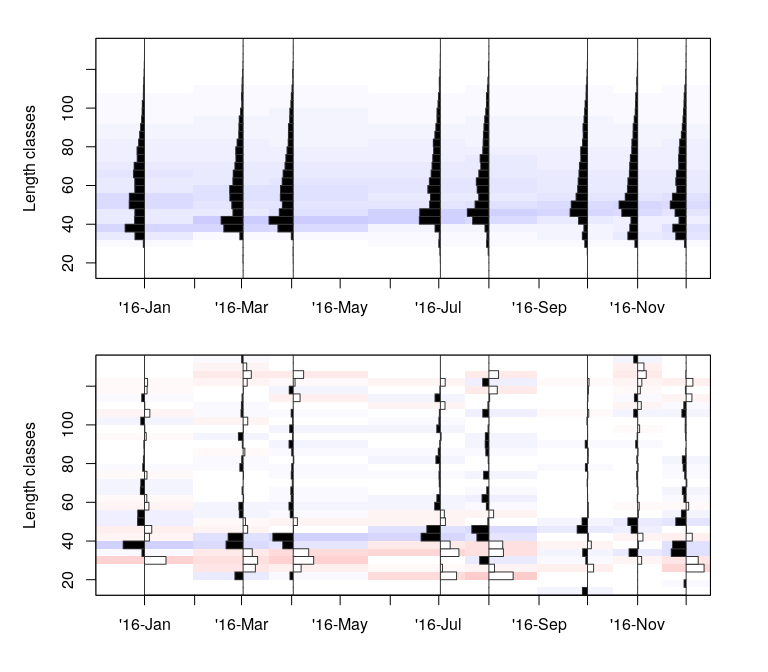Length frequency data visualised in terms of (a) catches and (b) restructured data with MA = 7.

For synLFQ7, a bin size of 4 cm and a moving average of 5 seems appropriate and will be used in the following. To get a first estimate of $$L_{inf}$$, the Powell-Wetherall method (Wetherall, Polovina, and Ralston 1987) can be applied. The method requires a catch vetor per length class representative for the length distribution in yearly catches instead of the catch matrix. The argument catch_columns allows to choose the columns of the catch matrix which will be summarised for the analysis. Here all columns are used as the catch matrix only includes catches from 2016. If data of several years are available, the data can be aggregated yearly and the results can be averaged or the data of several years is analysed jointly assuming constant growth parameters.

# Powell Wetherall plot
res_PW <- powell_wetherall(param = synLFQ7a,
catch_columns = 1:ncol(synLFQ7a$catch), reg_int = c(10,28)) # show results paste("Linf =",round(res_PW$Linf_est), "±", round(res_PW$se_Linf)) #>  "Linf = 132 ± 2"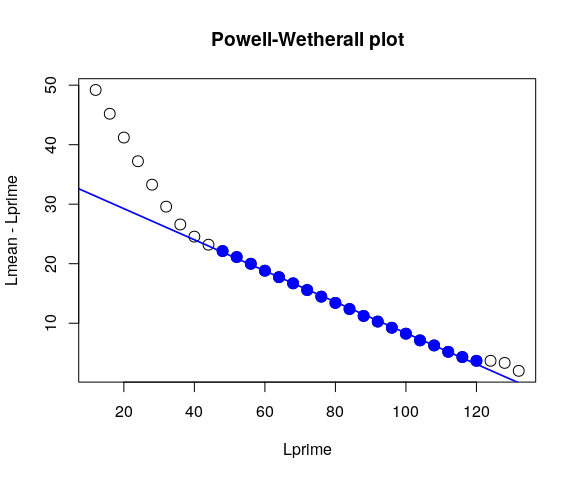Powell-Wetherall plot to derive an estimate of Linf. The argument reg_int is necessary in this tutorial because the “powell_wetherall” function includes an interactive plotting function where points for the regression analysis have to be selected by the user. Typically, one would not use this argument and instead choose which points to include in the regression analysis by clicking on the interactive plot (for more information see help(powell_wetherall)). For the data of this exercise the Powell-Wetherall method returns a $$L_{inf}$$ (± standard error) of 131.92 ± 1.77 cm, as determined by the x-intercept of the regression line. This estimate can be used for further analysis with ELEFAN. In TropFishR, there are 4 different methods based on the ELEFAN functionality: (i) K-Scan for the estimation of K for a fixed value of $$L_{inf}$$, (ii) Response Surface Analysis (RSA), (iii) ELEFAN with simulated annealing (“ELEFAN_SA”), and (iv) ELEFAN with a genetic algorithm (“ELEFAN_GA”), where the last three methods all allow to estimate K and $$L_{inf}$$ simultaneously. To get a quick K value corresponding to the $$L_{inf}$$ estimate of the Powell-Wetherall method, the estimate can be assigned to the argument Linf_fix in the function “ELEFAN”: # ELEFAN with K-Scan res_KScan <- ELEFAN(synLFQ7a, Linf_fix = res_PW$Linf_est,
MA=5, addl.sqrt = TRUE, hide.progressbar = TRUE)

# show results
res_KScan$par; res_KScan$Rn_max

This method, however, does not allow to test if different combinations of $$L_{inf}$$ and K might result in a better fit. RSA with a range around the $$L_{inf}$$ estimate from the Powell-Wetherall method can be used to check different combinations. Alternatively, the maximum length in the data or the maxmimum length class1 might be used as an reference for the search space of $$L_{inf}$$ (Taylor 1958; Beverton 1963). For this data set we chose a conservative range of the estimate from the Powell-Wetherall method plus/minus 10 cm. Any range can be chosen, while a larger search space increases computing time but gives a better overview of the score over a wide range of $$L_{inf}$$ and K combinations. A K range from 0.01 to 2 is relatively wide and should generally be sufficient.

# Response surface analyss
res_RSA <- ELEFAN(synLFQ7a, Linf_range = seq(119,139,1), MA = 5,
K_range = seq(0.01,2,0.1), addl.sqrt = TRUE,
hide.progressbar = TRUE, contour=5)

# show results
res_RSA$par; res_RSA$Rn_max

It is generally not recommendable to settle with the first estimate from RSA, as the method might find many local optima with close score values, but returns only the estimates associated with the highest score value. I recommend analysing several local maxima of the score function with a finer resolution for both parameters and compare the calculated score values and fit graphically. For this data, this automated procedure (code below) returns the highest score value (0.781) for the parameters $$L_{inf}$$ = 122.2, K = 0.21, and $$t_{anchor}$$ = 0.38 (more information on $$t_{anchor}$$ further down).

# find 3 highest score values
n <- length(res_RSA$score_mat) best_scores <- sort(res_RSA$score_mat,partial=n-0:2)[n-0:2]
ind <- arrayInd(which(res_RSA$score_mat %in% best_scores), dim(res_RSA$score_mat))
Ks <- as.numeric(rownames(res_RSA$score_mat)[ind[,1]]) Linfs <- as.numeric(colnames(res_RSA$score_mat)[ind[,2]])

res_loop <- vector("list", 3)
for(i in 1:3){
tmp <- ELEFAN(synLFQ7a,
Linf_range = seq(Linfs[i]-2, Linfs[i]+2, 0.2),
K_range = seq(Ks[i]-0.1, Ks[i]+0.1, 0.05),
MA = 5,
addl.sqrt = TRUE,
hide.progressbar = TRUE,
contour=5)
res_loop[[i]] <- cbind(Rn_max=tmp$Rn_max, t(as.matrix(tmp$par)))
}
results <- do.call(rbind, res_loop)

Note that RSA does not allow to optimise over the parameters C and $$t_s$$ of the seasonalised VBGF (soVBGF). It only allows to compare the score of ELEFAN runs with manually fixed C and $$t_s$$ values. In contrast, the newly implemented ELEFAN method ELEFAN_SA using a simulated annealing algorithm (Xiang et al. 2013) and ELEFAN_GA using genetic algorithms allow for the optimisation of the soVBGF (Taylor and Mildenberger 2017). The optimisation procedure in the simulated annealing algorithm gradually reduces the stochasticity of the search process as a function of the decreasing “temperature” value, which describes the probability of accepting worse conditions. In reference to the results of the Powell-Wetherall plot a second search within the range of 132 ± 10 cm for $$L_{inf}$$ is conducted. The search space of K is limted by 0.01 and 1.

# run ELEFAN with simulated annealing
res_SA <- ELEFAN_SA(synLFQ7a, SA_time = 60*0.5, SA_temp = 6e5,
MA = 5, seasonalised = TRUE, addl.sqrt = FALSE,
init_par = list(Linf = 129, K = 0.5, t_anchor = 0.5, C=0.5, ts = 0.5),
low_par = list(Linf = 119, K = 0.01, t_anchor = 0, C = 0, ts = 0),
up_par = list(Linf = 139, K = 1, t_anchor = 1, C = 1, ts = 1))
# show results
res_SA$par; res_SA$Rn_max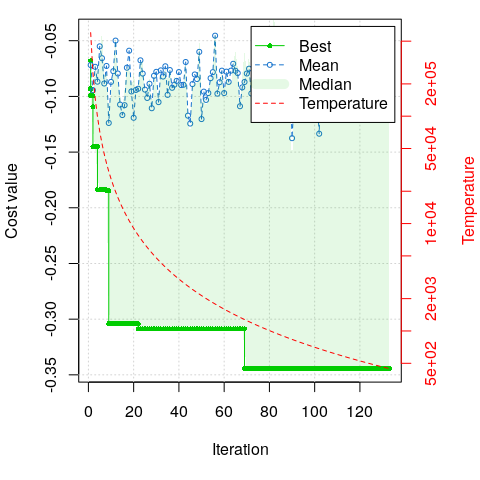Score graph of the ELEFAN method with simulated annealing. Green dots indicate the runnning minimum value of the cost function, while blue dots indicate the mean score of each iteration. The red line shows the decline of the ‘temperature’ value, which describes the probability of accepting worse solutions as the parameter space is explored.

Note that the computing time can be controlled with the argument “SA_time” and the results might change when increasing the time, in case the stable optimum of the objective function was not yet reached2. Due to the limitations of the vignette format the computation time was set to 0.5 minutes, which results already in acceptable results of $$L_{inf}$$ = 120.57, K = 0.23, $$t_{anchor}$$ = 0.4, C = 0.43, and $$t_s$$ = 0.97 with a score value ($$Rn_{max}$$) of 0.34. I recommend to increase ‘SA_time’ to 3 - 5 minutes to increase chances of finding the stable optimum. The jack knife technique allows to estimate a confidence interval around the parameters of the soVBGF (Quenouille 1956; Tukey 1958, 1962). This can be automated in R with following code:

JK <- vector("list", length(synLFQ7a$dates)) for(i in 1:length(synLFQ7a$dates)){
loop_data <- list(dates = synLFQ7a$dates[-i], midLengths = synLFQ7a$midLengths,
catch = synLFQ7a$catch[,-i]) tmp <- ELEFAN_SA(loop_data, SA_time = 60*0.5, SA_temp = 6e5, MA = 5, addl.sqrt = TRUE, init_par = list(Linf = 129, K = 0.5, t_anchor = 0.5, C=0.5, ts = 0.5), low_par = list(Linf = 119, K = 0.01, t_anchor = 0, C = 0, ts = 0), up_par = list(Linf = 139, K = 1, t_anchor = 1, C = 1, ts = 1), plot = FALSE) JK[[i]] <- unlist(c(tmp$par,list(Rn_max=tmp$Rn_max))) } JKres <- do.call(cbind, JK) # mean JKmeans <- apply(as.matrix(JKres), MARGIN = 1, FUN = mean) # confidence intervals JKconf <- apply(as.matrix(JKres), MARGIN = 1, FUN = function(x) quantile(x, probs=c(0.025,0.975))) JKconf <- t(JKconf) colnames(JKconf) <- c("lower","upper") # show results JKconf Depending on the number of sampling times (columns in the catch matrix) and the “SA_time”, this loop can take some time as ELEFAN runs several times, each time removing the catch vector of one of the sampling times. Another new optimisation routine is based on generic algorithms and is applied by: # run ELEFAN with genetic algorithm res_GA <- ELEFAN_GA(synLFQ7a, MA = 5, seasonalised = TRUE, maxiter = 50, addl.sqrt = FALSE, low_par = list(Linf = 119, K = 0.01, t_anchor = 0, C = 0, ts = 0), up_par = list(Linf = 139, K = 1, t_anchor = 1, C = 1, ts = 1), monitor = FALSE) # show results res_GA$par; res_GA$Rn_max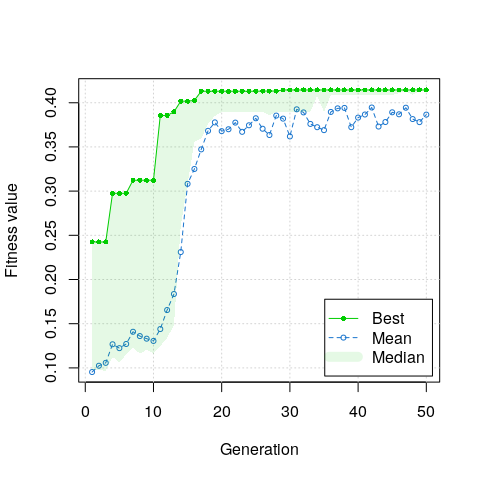Score graph of the ELEFAN method with genetic algorithms. Green dots indicate the runnning maximum value of the fitness function, while blue dots indicate the mean score of each iteration. The generation number of the ELEFAN_GA was set to only 50 generations (argument ‘maxiter’), which returns following results: $$L_{inf}$$ = 122.31, K = 0.22, $$t_{anchor}$$ = 0.43, C = 0.2, and $$t_s$$ = 0.5 with a score value ($$Rn_{max}$$) of 0.41. As with ELEFAN_SA the generation number was hold down due to the vignette format and should be increased in order to find more stable results. According to (Pauly 1980) it is not possible to estimate $$t_{0}$$ (theoretical age at length zero) from LFQ data alone. However, this parameter does not influence results of the methods of the traditional stock assessment workflow (catch curve, VPA/CA, and yield per recruit model) and can be set to zero (Mildenberger, unpublished). The ELEFAN methods in this package do not return starting points as FiSAT II users might be used to. Instead, they return the parameter “t_anchor”, which describes the fraction of the year where yearly repeating growth curves cross length equal to zero; for example a value of 0.25 refers to April 1st of any year. The maximum age is estimated within the ELEFAN function: it is the age when length is 0.95 $$L_{inf}$$. However, this value can also be fixed with the argument “agemax”, when alternative information about the maximum age of the fish species is available. The fit of estimated growth parameters can also be explored visually and indicates high similarity with true growth curves and a good fit through the peaks of the LFQ data. # plot LFQ and growth curves plot(lfqbin, Fname = "rcounts",date.axis = "modern", ylim=c(0,130)) lt <- lfqFitCurves(synLFQ7a, par = list(Linf=123, K=0.2, t_anchor=0.25, C=0.3, ts=0), draw = TRUE, col = "grey", lty = 1, lwd=1.5) # lt <- lfqFitCurves(synLFQ7, par = res_RSA$par,
#                    draw = TRUE, col = "goldenrod1", lty = 1, lwd=1.5)
lt <- lfqFitCurves(synLFQ7a, par = res_SA$par, draw = TRUE, col = "darkblue", lty = 1, lwd=1.5) lt <- lfqFitCurves(synLFQ7a, par = res_GA$par,
draw = TRUE, col = "darkgreen", lty = 1, lwd=1.5)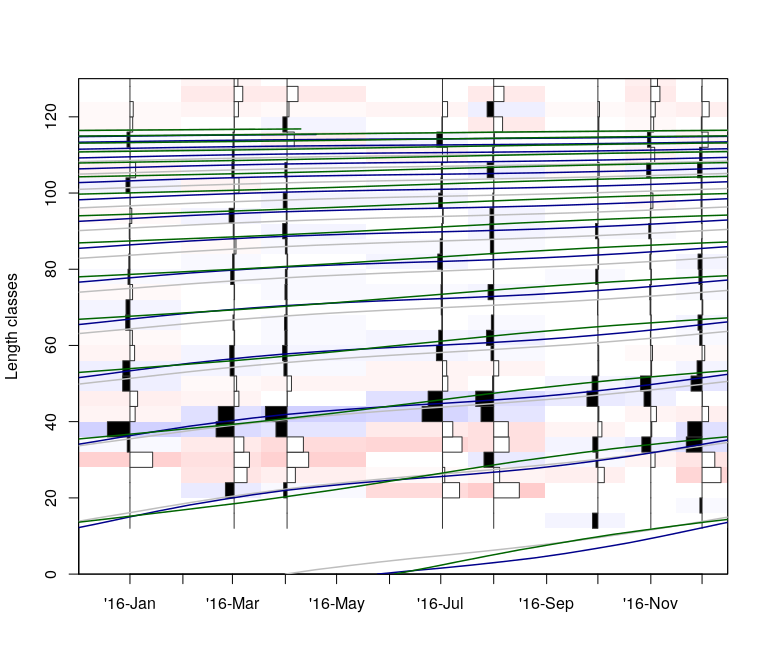Graphical fit of estimated and true growth curves plotted through the length frequency data. The growth curves with the true values are displayed in grey, while the blue and green curves represent the curves of ELEFAN_SA and ELEFAN_GA, respectively.

For further analysis, we use the outcomes of the simulated annealing approach by adding them to the Thumbprint Emperor data list.

# assign estimates to the data list
synLFQ7a <- c(synLFQ7a, res_SA$par) class(synLFQ7a) <- "lfq" ####Natural mortality The instantaneous natural mortality rate (M) is an influential parameter of stock assessment models and its estimation is challenging (Kenchington 2014; Powers 2014). When no controlled experiments or tagging data is available the main approach for its estimation is to use empirical formulas. Overall, there are at least 30 different empirical formulas for the estimation of this parameter (Kenchington 2014) relying on correlations with life history parameters and/or environmental information. We apply the most recent formula, which is based upon a meta-analysis of 201 fish species (Then et al. 2015). This method requires estimates of the VBGF growth parameters ($$L_{inf}$$ and K; Then et al. 2015). # estimation of M Ms <- M_empirical(Linf = res_SA$par$Linf, K_l = res_SA$par$K, method = "Then_growth") synLFQ7a$M <- as.numeric(Ms)
# show results
paste("M =", as.numeric(Ms))
#>  "M = 0.286"

The result is a natural mortality of 0.29 year$$^{-1}$$.

###Fisheries aspects ####Exploitation level In order to estimate the level of exploitation, knowledge on fishing mortality (F) (usually derived by subtracting natural mortality from total mortality) and gear selectivity is necessary. The length-converted catch curve allows the estimation of the instantaneous total mortality rate (Z) of LFQ data and the derivation of a selection ogive. Here we skip an in-depth selectivity exploration, because more data would be required for this assessment3. The following approach assumes a logistic selection ogive, typical for trawl-net selectivity, which may provide an appropriate first estimate in the case of LFQ data derived from a mixture of gears. Total mortality rate is estimated with a sample of the catch representative for the whole year. Besides, changing the bin size, the function lfqModify allows to rearrange the catch matrix in the required format (catch vector per year) and to pool the largest length classes with only a few individuals into a plus group (necessary later for the cohort analysis). As with the Powell-Wetherall method, the reg_int argument is necessary to avoid the interactive plotting function (more information in help(catchCurve)). The argument calc_ogive allows the estimation of the selection ogive.

# summarise catch matrix into vector and add plus group which is smaller than Linf
synLFQ7b <- lfqModify(synLFQ7a, vectorise_catch = TRUE, plus_group = 118)
# run catch curve
res_cc <- catchCurve(synLFQ7b, reg_int = c(8,26), calc_ogive = TRUE)
# assign estimates to the data list
synLFQ7b$Z <- res_cc$Z
synLFQ7b$FM <- as.numeric(synLFQ7b$Z - synLFQ7b$M) synLFQ7b$E <- synLFQ7b$FM/synLFQ7b$Z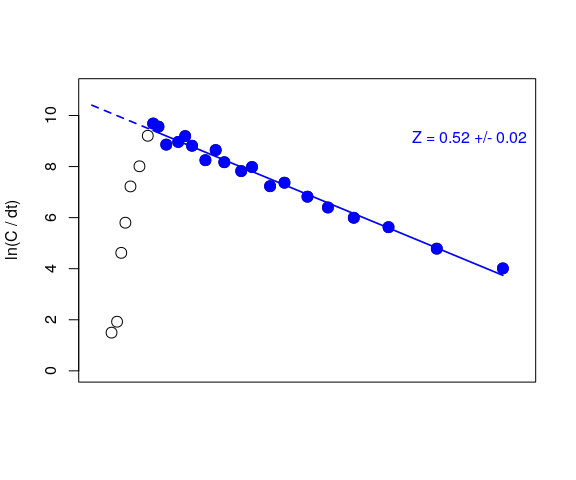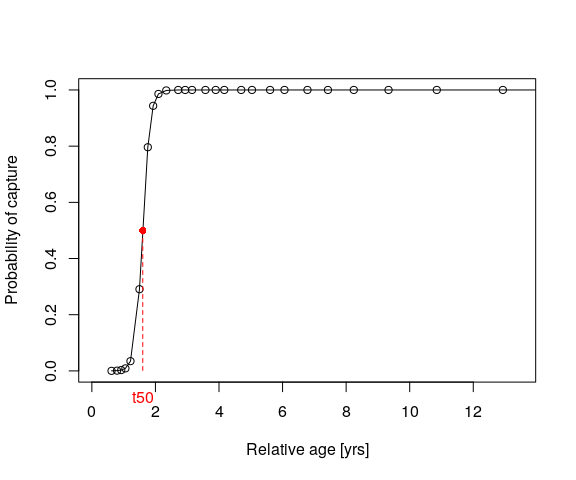#>  "Z = 0.52"
#>  "FM = 0.23"
#>  "E = 0.44"
#>  "L50 = 36.56"

The catch curve analysis returns a Z value of 0.52 $$year^{-1}$$. By subtracting M from Z, the fishing mortality rate is derived: 0.23 $$year^{-1}$$. The exploitation rate is defined as $$E = F/Z$$ and in this example 0.44 The selectivity function of the catch curve estimated a length at first capture ($$L_{50}$$) of 36.56 cm.

###Stock size and status ####Stock size and composition The stock size and fishing mortality per length class can be estimated with Jones’ length converted cohort analysis (CA, Jones 1984) - a modification of Pope’s virtual population analysis (VPA) for LFQ data. It requires the estimates from preceeding analysis and in addition the parameters a and b of the allometric length-weight relationship4. Furthermore, CA needs an estimate for the terminal fishing mortality (terminal_F), which was set here to the result of the catch curve minus natural mortality (0.235). The cohort analysis estimates the stock size based on the total catches, it is therefore necessary that the catch vector is representative for the full stock and for all fisheries catches targeting this stock. The argument “catch_corFac” can be used to raise the catches to be yearly or spatially representative. Here I assume that all fisheries targeting the stock were sampled and the catch during the four missing months corresponds to the average monthly catch (catch_corFac = (1 + 4/12)). The use of the function lfqModify with the argument “plus_group” is necessary as CA does not allow length classes larger than $$L_{inf}$$. If the argument “plus_group” is set to TRUE only, the function shows the catches per length class and asks the user to enter a length class corresponding to the length class of the new “plus group”. If “plus_group” is set to a numeric (here 122, which is just below $$L_{inf}$$), the plus group is created at this length class (numeric has to correspond to existing length class in vector “midLengths”).

synLFQ7c <- synLFQ7b

# assign length-weight parameters to the data list
synLFQ7c$a <- 0.015 synLFQ7c$b <- 3
# run CA
vpa_res <- VPA(param = synLFQ7c, terminalF = synLFQ7c$FM, analysis_type = "CA", plot=TRUE, catch_corFac = (1+4/12)) # stock size sum(vpa_res$annualMeanNr, na.rm =TRUE) / 1e3
#>  342.5927
# stock biomass
sum(vpa_res$meanBiomassTon, na.rm = TRUE) #>  1186801 # assign F per length class to the data list synLFQ7c$FM <- vpa_res$FM_calc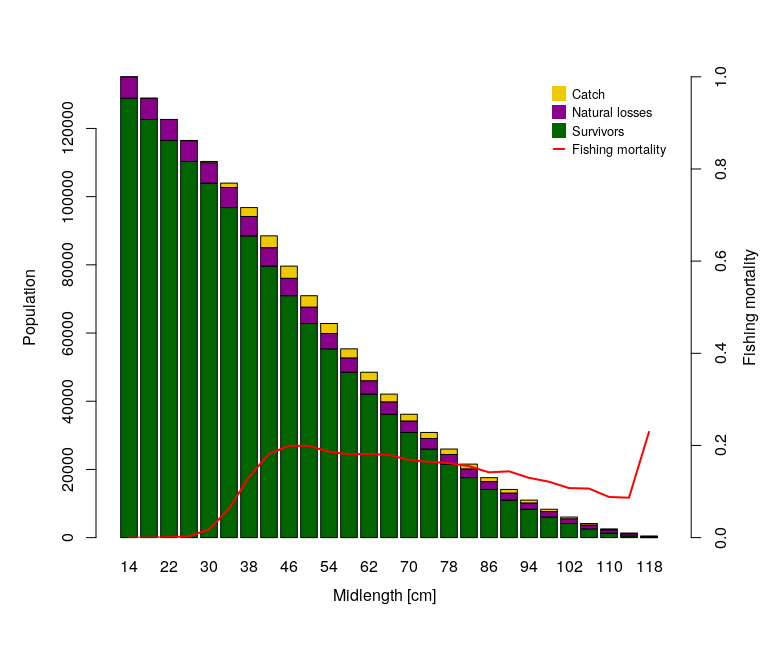Results of Jones’ cohort analysis (CA). The results show the logistic shaped fishing pattern across length classes (red line in CA plot). The size of the stock is returned in numbers and biomass and according to this method 3.4259310^{5} individuals and 1.18680110^{6} tons, respectively. ####Yield per recruit modelling Prediction models (or per-recruit models, e.g. Thompson and Bell model) allow to evaluate the status of a fish stock in relation to reference levels and to infer input control measures, such as restricting fishing effort or regulating gear types and mesh sizes. By default the Thompson and Bell model assumes knife edge selection ($$L_{25}$$ = $$L_{50}$$ = $$L_{75}$$)6; however, the parameter s_list allows for changes of the selectivity assumptions. The parameter FM_change determines the range of the fishing mortality for which to estimate the yield and biomass trajectories. In the second application of this model, the impact of mesh size restrictions on yield is explored by changing $$L_{c}$$ (Lc_change) and F (FM_change, or exploitation rate, E_change) simultaneously. The resulting estimates are presented as an isopleth graph showing yield per recruit. By setting the argument stock_size_1 to 1, all results are per recruit. If the number of recruits (recruitment to the fishery) are known, the exact yield and biomass can be estimated. The arguments curr.E and curr.Lc allow to derive and visualise yield and biomass (per recruit) values for current fishing patterns. # Thompson and Bell model with changes in F TB1 <- predict_mod(synLFQ7c, type = "ThompBell", FM_change = seq(0,1.5,0.05), stock_size_1 = 1, curr.E = synLFQ7c$E, plot = FALSE, hide.progressbar = TRUE)
# Thompson and Bell model with changes in F and Lc
TB2 <- predict_mod(synLFQ7c, type = "ThompBell",
FM_change = seq(0,1.5,0.1), Lc_change = seq(25,50,0.1),
stock_size_1 = 1,
curr.E = synLFQ7c$E, curr.Lc = res_cc$L50,
s_list = list(selecType = "trawl_ogive",
L50 = res_cc$L50, L75 = res_cc$L75),
plot = FALSE, hide.progressbar = TRUE)
# plot results
par(mfrow = c(2,1), mar = c(4,5,2,4.5), oma = c(1,0,0,0))
plot(TB1, mark = TRUE)
mtext("(a)", side = 3, at = -1, line = 0.6)
plot(TB2, type = "Isopleth", xaxis1 = "FM", mark = TRUE, contour = 6)
mtext("(b)", side = 3, at = -0.1, line = 0.6)

# Biological reference levels
TB1$df_Es #> Fmsy F05 Emsy E05 #> 1 0.45 0.2 0.611413 0.4115226 # Current yield and biomass levels TB1$currents
#>   curr.Lc curr.tc    curr.E    curr.F    curr.C   curr.Y curr.V   curr.B
#> 1      NA      NA 0.4449351 0.2292551 0.2749782 1253.466      0 8781.828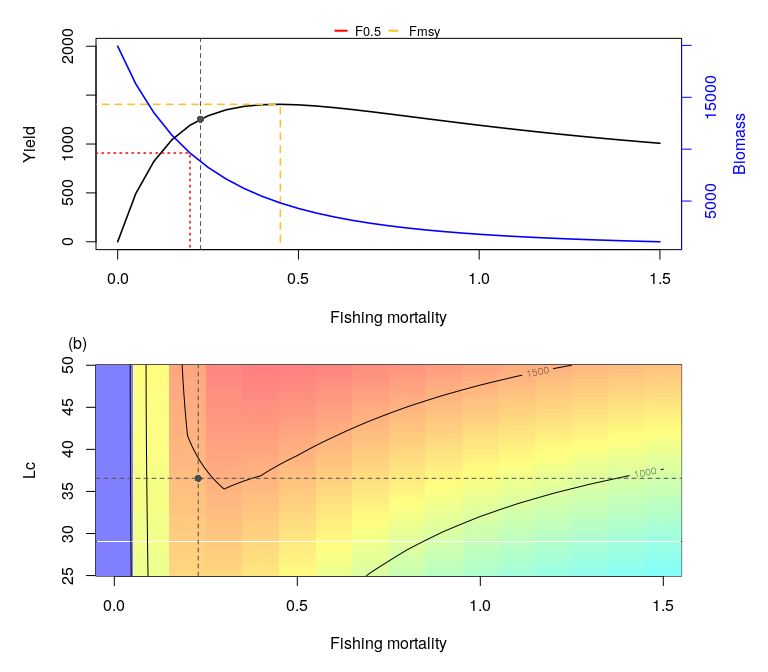Results of the Thompson and Bell model: (a) Curves of yield and biomass per recruit. The black dot represents yield and biomass under current fishing pressure. The yellow and red dashed lines represent fishing mortality for maximum sustainable yield (Fmsy) and fishing mortality to fish the stock at 50% of the virgin biomass (F0.5). (b) exploration of impact of different exploitation rates and Lc values on the relative yield per recruit.

Please note that the resolution of the $$L_c$$ and F changes is quite low and the range quite narrow due to the limitations in computation time of the vignette format. The results indicate that the fishing mortality of this example (F = 0.23) is higher than the maximum fishing mortality ($$F_{max} =$$ 0.45), which confirms the indication of the slightly increased exploitation rate (E = 0.44). The prediction plot shows that the yield could be increased when fishing mortality and mesh size is increased. The units are grams per recruit.

##Summary For management purposes, fish stock assessments are mainly conducted for single species or stocks, which describe the manamgent units of a population. There is much to be gained from multi-species and ecosystem models, but data requirements and complexity make them often unsuitable for deriving management advice. For data-poor fisheries, a traditional fish stock assessment solely based on length-frequency (LFQ) data of one year (as presented here) is particularly useful. LFQ data comes with many advantages over long time series of catch and effort or catch-at-age data (Mildenberger, Taylor, and Wolff 2017). In this exercise, the exploitation rate and results of the yield per recruit models indicate that the fiseries is close to sustainable exploitation. The exploration of stock status and fisheries characteristics can of course be extended, but go beyond the scope of this tutorial, which is thought to help getting started with the TropFishR package. Further details about functions and their arguments can be found in the help files of the functions (help(...) or ?.., where the dots refer to any function of the package). Also the two publications by Mildenberger, Taylor, and Wolff (2017) and by Taylor and Mildenberger (2017) provide more details about the functionality and context of the package.

##Author’s comment If you have comments or questions please write an email or post an issue at GitHub. You can follow the development of TropFishR on ResearchGate.

##References

Beverton, R. J. 1963. “Maturation, growth and mortality of clupeid and engraulid stocks in relation to fishing.” Rapport Conseil International Exploration de La Mer 154: 44–67.

Hilborn, Ray, and Carl J. Walters. 1992. Quantitative Fisheries Stock Assessment. Vol. 1. https://doi.org/10.1017/CBO9781107415324.004.

Jones, R. 1984. “Assessing the effects of changes in exploitation pattern using length composition data (with notes on VPA and cohort analysis).” FAO Fisheries Technical Paper, no. 256: 118p.

Kenchington, Trevor J. 2014. “Natural mortality estimators for information-limited fisheries.” Fish and Fisheries 15 (4): 533–62. https://doi.org/10.1111/faf.12027.

Mildenberger, Tobias Karl, Marc Hollis Taylor, and Matthias Wolff. 2017. “TropFishR: An R Package for Fisheries Analysis with Length-Frequency Data.” Methods in Ecology and Evolution 8 (11): 1520–7. https://doi.org/10.1111/2041-210X.12791.

Millar, R. B., and R. Holst. 1997. “Estimation of gillnet and hook selectivity using log-linear models.” ICES Journal of Marine Science 54 (1963): 471–77. https://doi.org/10.1006/jmsc.1996.0196.

Pauly, Daniel. 1980. “On the Interrelationships Between Natural Mortality, Growth Parameters, and Mean Environmental Temperature in 175 Fish Stocks.” https://doi.org/10.1093/icesjms/39.2.175.

Powers, J. E. 2014. “Age-specific natural mortality rates in stock assessments: size-based vs. density-dependent.” ICES Journal of Marine Science: Journal Du Conseil 71 (7): 1629–37.

Quenouille, M. H. 1956. “Notes on bias in estimation.” Biometrika 43: 353–60.

Sparre, P., and SC Venema. 1998. “Introduction to Tropical Fish Stock Assessment.” FAO Technical Paper.

Taylor, C. C. 1958. “Cod growth and temperature.” Journal Du Conseil 23 (3): 366–70.

Taylor, M. H., and T. K. Mildenberger. 2017. “Extending Electronic Length Frequency Analysis in R.” Fisheries Management and Ecology 24 (4): 330–38. https://doi.org/10.1111/fme.12232.

Then, Amy Y., John M. Hoenig, Norman G. Hall, and David A. Hewitt. 2015. “Evaluating the prodicitve performance of emiprical estimators of natural mortality rate using information on over 200 fish species.” ICES Journal of Marine Science 72 (1): 82–92. https://doi.org/10.1093/icesjms/fst034.

Tukey, J. 1958. “Bias and confidence in not quite large samples.” Annals of Mathematical Statistics 29: 614.

Tukey, John W. 1962. “The Future of Data Analysis.” The Annals of Mathematical Statistics 33 (1): 1–67.

Wetherall, J. A., J. J. Polovina, and S. Ralston. 1987. “Estimating growth and mortality in steady-state fish stocks from length-frequency data.” ICLARM Conference Proceedings 13: pp. 53–74.

Xiang, Y, Sylvain Gubian, Brian Suomela, and Julia Hoeng. 2013. “Generalized simulated annealing for global optimization: the GenSA Package.” R Journal 5 (June): 13–28. http://rjournal.github.io/archive/2013-1/xiang-gubian-suomela-etal.pdf.

1. or average of the several largest lengths or length classes

2. Stable optimum is indicated by overlapping blue and green dots in the score graph.

3. For a comprehensive description of selectivity estimation refer to Millar and Holst (1997).

4. Here the true parameters of a = 0.015 and b = 3 are used assuming that this was calculated from length-weight data.

5. For a discussion on this parameter see Hilborn and Walters (1992)

6. Note that the length at capture has 2 abbreviations $$L_{50}$$ and $$L_c$$.